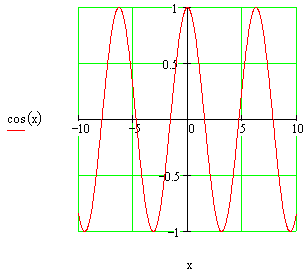# The Graph of the Cosine FunctionTo the left is the graph of the cosine function.

Click the EZ Graph link below to draw this graph with a graphic calculator, explanations included. Links for other examples of the cosine function graph along with their explanations follow below.

More graphs of the cosine function using EZ Graph:

Angle in degrees: